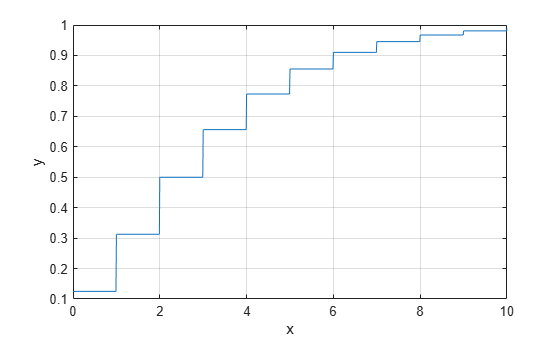# nbincdf

Negative binomial cumulative distribution function

## Syntax

```y = nbincdf(x,R,p) y = nbincdf(x,R,p,'upper') ```

## Description

`y = nbincdf(x,R,p)` computes the negative binomial cdf at each of the values in `x` using the corresponding number of successes, `R` and probability of success in a single trial, `p`. `x`, `R`, and `p` can be vectors, matrices, or multidimensional arrays that all have the same size, which is also the size of `y`. A scalar input for `x`, `R`, or `p` is expanded to a constant array with the same dimensions as the other inputs.

`y = nbincdf(x,R,p,'upper')` returns the complement of the negative binomial cdf at each value in `x`, using an algorithm that more accurately computes the extreme upper tail probabilities.

The negative binomial cdf is

`$y=F\left(x|r,p\right)=\sum _{i=0}^{x}\left(\begin{array}{c}r+i-1\\ i\end{array}\right){p}^{r}{q}^{i}{I}_{\left(0,1,...\right)}\left(i\right)$`

The simplest motivation for the negative binomial is the case of successive random trials, each having a constant probability `p` of success. The number of extra trials you must perform in order to observe a given number `R` of successes has a negative binomial distribution. However, consistent with a more general interpretation of the negative binomial, `nbincdf` allows `R` to be any positive value, including nonintegers. When `R` is noninteger, the binomial coefficient in the definition of the cdf is replaced by the equivalent expression

`$\frac{\Gamma \left(r+i\right)}{\Gamma \left(r\right)\Gamma \left(i+1\right)}$`

## Examples

collapse all

```x = (0:15); p = nbincdf(x,3,0.5); stairs(x,p)```